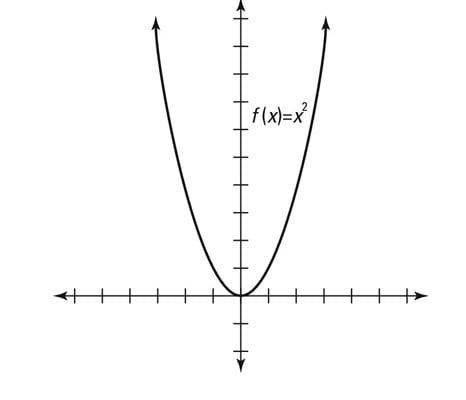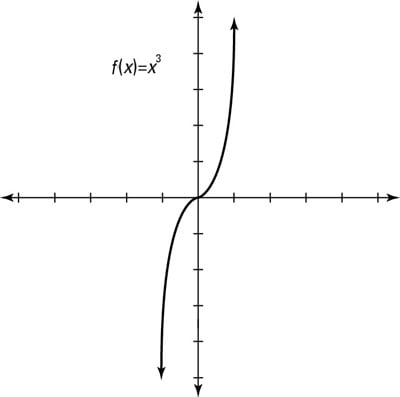##### Pre-Calculus Workbook For DummiesKnowing whether a function is even or odd helps you to graph it because that information tells you which half of the points you have to graph. These types of functions are symmetrical, so whatever is on one side is exactly the same as the other side.

If a function is even, the graph is symmetrical about the y-axis. If the function is odd, the graph is symmetrical about the origin.

• Even function: The mathematical definition of an even function is f(–x) = f(x) for any value of x. The simplest example of this is f(x) = x2 because f(x)=f(-x) for all x. For example, f(3) = 9, and f(–3) = 9. Basically, the opposite input yields the same output.Visually speaking, the graph is a mirror image about the y-axis, as shown here.
• Odd function: The definition of an odd function is f(–x) = –f(x) for any value of x. The opposite input gives the opposite output. These graphs have 180-degree symmetry about the origin. If you turn the graph upside down, it looks the same.The example shown above, f(x) = x3, is an odd function because f(-x)=-f(x) for all x. For example, f(3) = 27 and f(–3) = –27.